Name:    Chapter 7 Test

Multiple Choice
Identify the choice that best completes the statement or answers the question.

Find the volume.

1.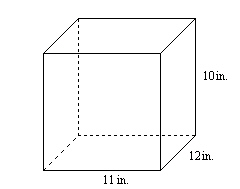a. 132 in.3 c. 480 in.3 b. 33 in.3 d. 1320 in.3

2.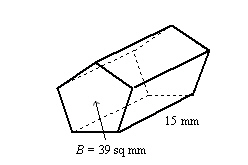a. 54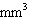c. 114b. 153d. 585Find the volume.

3.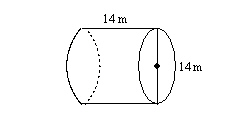a.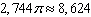m3 c.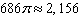m3 b.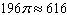m3 d.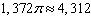m3

Find the volume.

4.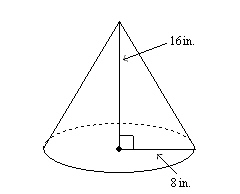a.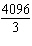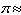4287.1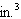c.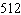1607.7b. 10243215.4d.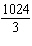1071.85.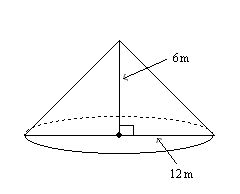a.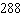904.3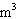c. 216678.2b.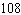339.1d.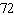226.1Find the volume.

6.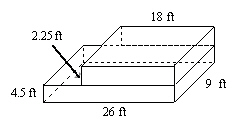a. 1053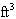c. 59.75b. 1579.5d. 1417.57.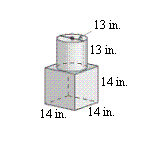a. 68c. 5,292b. 2,744 +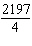4,468.6d. 2,744 + 2,1979,642.68.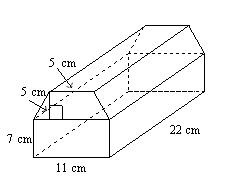a. 50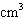c. 2,244b. 2,904d. 2,574Numeric Response

1.

A cereal box is 15 inches by 10 inches by 3 inches. What is the volume of the box?

Determine whether the solids are similar.

1.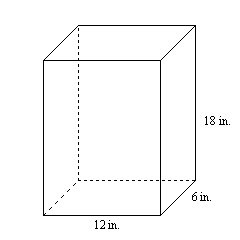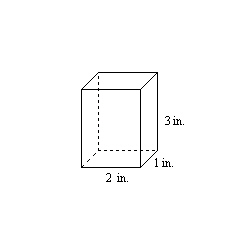2.

The ratio of the corresponding linear measures of two similar cans of fruit is 1 : 2. The smaller can has a surface area of 120 square centimeters and a volume of 225.6 cubic centimeters. Find the surface area and volume of the larger can.